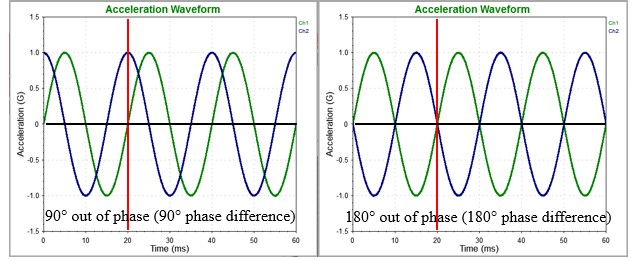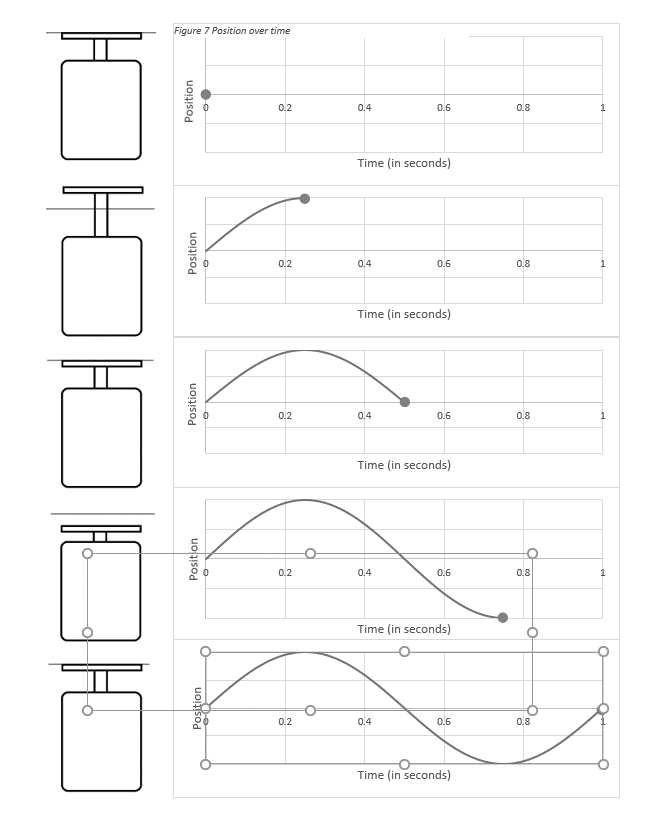# Phase of a Sine Wave

March 29, 2018

In the previous lesson, we learned about the frequency, period, and amplitude measurements of a sine wave. Phase is another measurement of the sine wave, and it indicates where the wave is in its cycle. It is measured in degrees (0°-360°) or radians (0-2π) and is denoted with the Greek symbol Phi (ϕ).

### Phase Comparison

In vibration testing, an engineer’s interest is not so much the phase of an individual wave but the phase difference between a sine wave and a reference wave. Two sine waves are out of phase when they are not at the same point in their cycle at the same time.Figure 1.4. The phase difference between two sine waves. The left is a 90° phase difference; the right is a 180° difference.

“90 degrees out of phase” means when one wave is at zero, the other will be at its peak (see Figure 1.4.) In other words, when the green wave is at 0° phase, the blue wave is at 90°.

“180 degrees out of phase” means the zero points remain the same, but when one signal is at its peak, the other is at its trough. In other words, when the green wave is at 0° phase, the blue wave is at 180°.

### Sine Vibration Testing

Now, we can apply frequency, period, amplitude, and phase to vibration testing. When an engineer runs a sine test on a shaker at a fixed frequency and amplitude, the shaker head oscillates at a regular frequency and amplitude.

#### Example

Say we placed a device that measures position on a shaker head and ran a single-tone sine test at 1 hertz (Hz). The plot of the device’s position over time and relative to the rest position would look similar to Figure 1.5. The shaker head would complete one cycle over the course of one second. Similarly, if we ran a single-tone sine test at 500Hz, the shaker would complete 500 cycles in one second.Figure 1.5. The position of a device over time relative to the rest position.